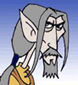Problem with scale controller expression

Why this script does not work?

for i in selection do
(
if classOf i == BoneGeometry then
(
b = box length:i.height \
width:i.length \
height:i.width \
pivot:[-i.length/2, 0, i.width/2]
b.transform = i.transform
b.parent = i
b.scale.controller = scale_script()
b.scale.controller.script = "\$.parent.stretchTM.scale"
--above is the line with problem
)
)

What am I doing wrong?
And thanks for any help.

Comment viewing optionsThat's correct, you should

That's correct, you should avoid using the "\$" sign in the script part of a scripted controller. Instead you should use the .addNode method (check the maxscript help on script controllers) to add a local variable for the controller and then assign that on the script string. somewhat like this (not tested, by the way)

b.scale.controller = scale_script()
b.scale.script = "obj.parent.stretchTM.scale"

This will throw an error if the node doesn't have a parent, so you should add some extra checking in your script before assigning the script controllerThis sign "\$" means the

This sign "\$" means the object need to be selected, and if more than one object is selected also will not works, so shortly use the object name instead, i.e.:

-- replace this line...
b.scale.controller.script = "\$.parent.stretchTM.scale"
-- with this one...
b.scale.controller.script = "\$"+b.name+".parent.stretchTM.scale"

my recent MAXScripts RSS (archive here)